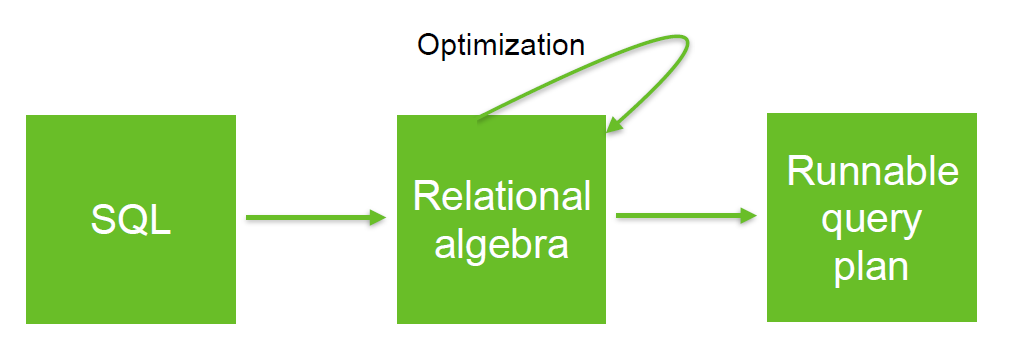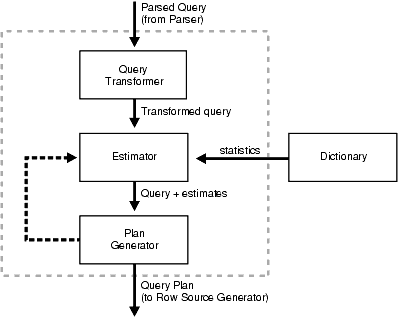# Algebraic Optimization

## Example

$$N = ((Z*2)+((Z*3)+0))/1$$

1. (+) identity: x+0 = x
2. (/) identity: x/1 = x
3. (*) distributes: (n*x+n*y) = n*(x+y)
4. (*) commutes: x*y = y*x

Apply rules 1,3,4,2 and we get $$N = (2+3)*z$$

Two operations instead of five, no division operator

Same idea works with the Relational Algebra

## Filter

### Filter early

Filter Early is an algebraic optimization:

### Predicate push Down

Predicate push Down is the same that filter early.

It is valid to push a filter into an input of an inner join if the filter does not reference columns from the other input.

## Others

• Column pruning

## No Sort

Example from CALCITE-873

Given the following table:

CREATE TABLE T (
K1 VARCHAR,
K2 VARCHAR,
K3 VARCHAR,
CONSTRAINT pk PRIMARY KEY (K1, K2, K3));


In the following queries, no sort is necessary:

SELECT * FROM T WHERE K1='A' ORDER BY K2,K3;
SELECT * FROM T WHERE K2='B' ORDER BY K1,K3;
SELECT * FROM T WHERE K1='A' AND K2='B' ORDER BY K3;


## Documentation / Reference

Discover MoreCalcite - Optimizer (RelOptCluster)

The optimizer is a program that takes a relational expression (query plan) and rewrites it with optimization rules. The output is still a relational expression and is generally called the physical plan....Data Partition - Partition Pruning (Elimination)

Partition Pruning is access paths methods that occurs when a table is partitioned by the column in the predicate. In this case, the database will read only the partitions involved and not the full table....Relational Algebra - Expression and Operators

Relational algebra is based upon the fact that you can pass tabular data through a set of data operators (select, filter, join, sort, union, etc.) in a algebraic structure. It means that: the output...SQL Engine - Query Optimizer (Query Optimization)

in a SQL Engine. A SQL statement can be executed in many different ways, such as: full table scans, index scans, nested loops, hash joins. The query optimizer determines the most efficient...